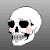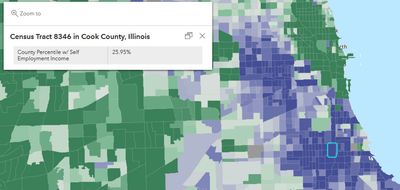# Calculate percentile with Arcade expression for pop up

245
2
07-23-2021 04:37 PM
Labels (1)New Contributor II

Hi all!

Does anyone know how to calculate a percentile using an arcade string for a pop-up in ArcGIS Online?

I am working on data for Glynn county Georgia and have percentages such as percent minority for each census block. I would like for the pop up to display the percentile that the block falls within the county: ex. this census block is in the 90th percentile for minority population.

Does anyone know how to write a script that does this? I am completely new to Arcade.

Tags (3)
1 Solution

Accepted SolutionsbyMVP Regular Contributor

This should be straightforward enough but does depend on the input data. I'll use an ACS household income layer in my example. In particular, I'm going to get the percentile rank of a given census tract based on the attribute B19053_002E, which is the number of households reporting self-employment income.

The formula for percentile for a value X is [number of values < X] / total number of values]. In an Arcade expression, it looks like the following. Note that I'm including a Filter function to only get percentiles for a single county.

``````// The value to get the percentile for
var value = \$feature["B19053_002E"]

// The subset of the GEOID referring to state and county
// We insert the SQL wildcard "%" here, as the Filter parameter seems to have a problem with using it there
var county_id = Left(\$feature["GEOID"], 5) + "%"

// Census tracts. Only getting two relevant fields, no geometry.
var tracts = FeatureSetByName(
\$map,
"ACS Median Household Income Variables - Boundaries - Tract",
["GEOID", "B19053_002E"],
false)

// Filter tracts by matching county ID
var county_tracts = Filter(tracts, "GEOID LIKE @county_id")

// Filter again for values below clicked feature
var below_tracts = Filter(county_tracts, "B19053_002E < @value")

// Calculate percentile
var percentile = Count(below_tracts)/Count(county_tracts)
return Text(percentile, '#.00%')``````- Josh Carlson
Kendall County GIS
2 RepliesbyMVP Regular Contributor

This should be straightforward enough but does depend on the input data. I'll use an ACS household income layer in my example. In particular, I'm going to get the percentile rank of a given census tract based on the attribute B19053_002E, which is the number of households reporting self-employment income.

The formula for percentile for a value X is [number of values < X] / total number of values]. In an Arcade expression, it looks like the following. Note that I'm including a Filter function to only get percentiles for a single county.

``````// The value to get the percentile for
var value = \$feature["B19053_002E"]

// The subset of the GEOID referring to state and county
// We insert the SQL wildcard "%" here, as the Filter parameter seems to have a problem with using it there
var county_id = Left(\$feature["GEOID"], 5) + "%"

// Census tracts. Only getting two relevant fields, no geometry.
var tracts = FeatureSetByName(
\$map,
"ACS Median Household Income Variables - Boundaries - Tract",
["GEOID", "B19053_002E"],
false)

// Filter tracts by matching county ID
var county_tracts = Filter(tracts, "GEOID LIKE @county_id")

// Filter again for values below clicked feature
var below_tracts = Filter(county_tracts, "B19053_002E < @value")

// Calculate percentile
var percentile = Count(below_tracts)/Count(county_tracts)
return Text(percentile, '#.00%')``````- Josh Carlson
Kendall County GISNew Contributor II

Thank you so much!!!!